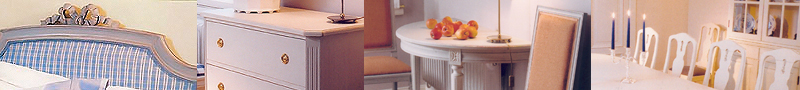P R O D U C T S C H A I R S  |   A R M C H A I R S  |   T A B O U R E T S  &  B E N C H E S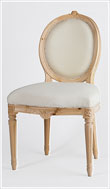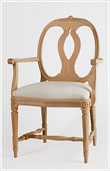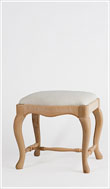G R I P S H O L M   F U R N I T U R E  |   B E D R O O M  |   M I R R O R S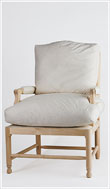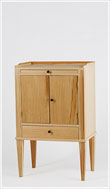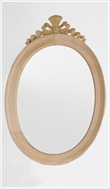C H E S T   O F   D R A W E R S  |   B U F F E T S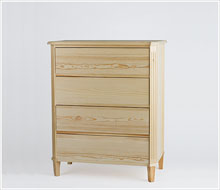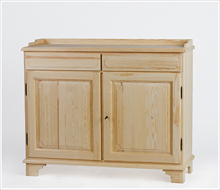W R I T I N G D E S K S  |   T A B L E S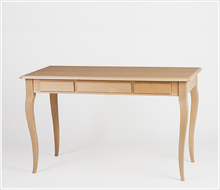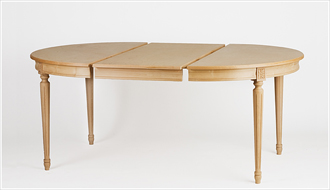C U P B O A R D S   &   B O O K C A S E SW A L L   C A B I N E T S    &   P L A T E   R A C K S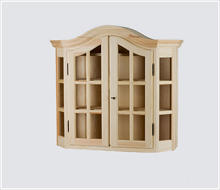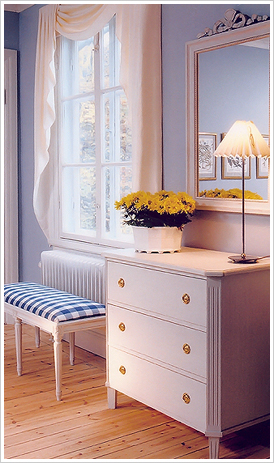© S O LARSSON STILMÖBLER EFTR. AB  |  E-MAIL: LARSSON.STILMOBLER@TELIA.COM  |  PRODUCTION: BÅS